Programming the Arduino
Part 3: Switches and buttons

Switches

A switch is an electrical component that changes the path of electricity through a circuit as it is moved between different physical positions. The particular switch in your kit is known as a “single-pole, dual-throw” (SPDT) switch because it switches a single circuit between two terminals.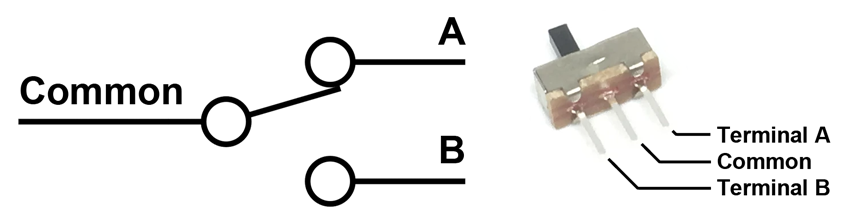DO THIS: Hooking up a switch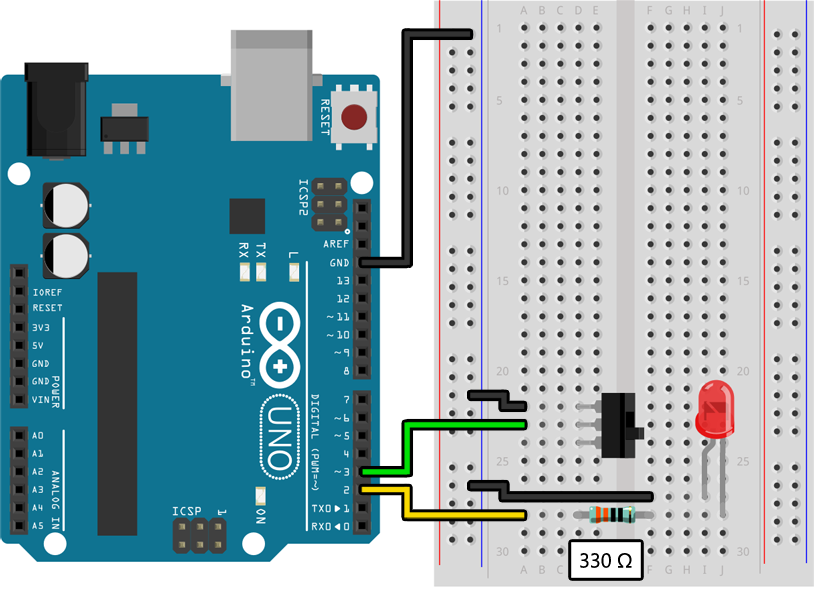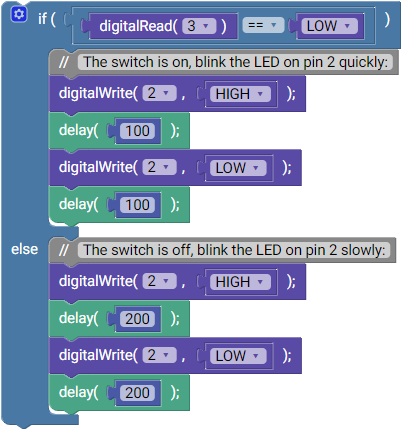``````void setup()
{
pinMode(2, OUTPUT);
pinMode(3, INPUT_PULLUP);
}

void loop()
{
{
// The switch is on, blink the LED on pin 2 quickly:
digitalWrite(2, HIGH);
delay(100);
digitalWrite(2, LOW);
delay(100);
}
else
{
// The switch is off, blink the LED on pin 2 slowly:
digitalWrite(2, HIGH);
delay(200);
digitalWrite(2, LOW);
delay(200);
}
}
``````

Your LED should be blinking at a rate determined by the position of the switch, with one position causing it to blink twice as fast as the other position.

Using pins as inputs

Just like in the previous lesson we are using `pinMode()` to configure pin 2 as an output that can turn an LED on and off: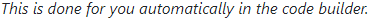``````pinMode(2, OUTPUT);
``````

We are also doing something new, however, and are hooking up a switch to pin 3 so that we can use it as an input. To do this we must set the pin mode to `INPUT_PULLUP`:``````pinMode(3, INPUT_PULLUP);
``````

To turn an output on and off we use `digitalWrite()` with the pin number and either `HIGH` (to turn it on) or `LOW` (to turn it off):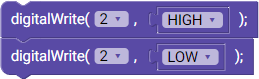``````digitalWrite(2, HIGH);
digitalWrite(2, LOW);
``````

Similarly, to read from an input, we use `digitalRead()` with the pin number and then test if it is equal to `HIGH` or `LOW` with an `if`/`else` statement: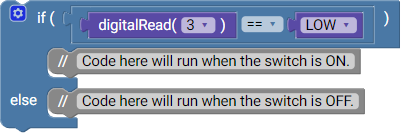``````if (digitalRead(3) == LOW)
{
// Code here will run when the switch is ON.
}
else
{
// Code here will run when the switch is OFF.
}
``````

DO THIS: Hooking up a button

In addition to switches, your kit also contains buttons, which are only active when held: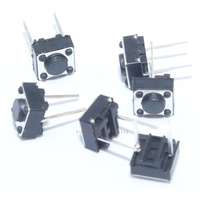Buttons can be connected to the Arduino in exactly the same way as switches. Try replacing the switch in the above example with a button as shown here: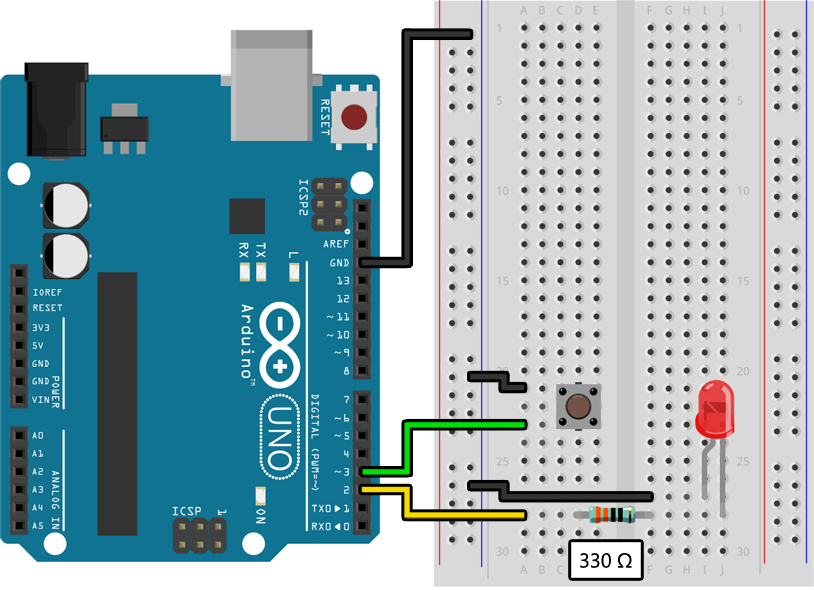If/else statements

The `if`/`else` statement in the above example has one block of code that runs when the condition `digitalRead(3) == LOW` is true and another block of code that runs when it is not:``````if (digitalRead(3) == LOW)
{
// The switch is on, blink the LED on pin 2 quickly:
digitalWrite(2, HIGH);
delay(100);
digitalWrite(2, LOW);
delay(100);
}
else
{
// The switch is off, blink the LED on pin 2 slowly:
digitalWrite(2, HIGH);
delay(200);
digitalWrite(2, LOW);
delay(200);
}
``````

If we simplify this code to just the `if`/`else` statement it looks like this:``````if (digitalRead(3) == LOW)
{
// Code here will run when the switch is ON.
}
else
{
// Code here will run when the switch is off.
}
``````

If you don't care about when the condition is not true, however, you can omit the `else` block like this: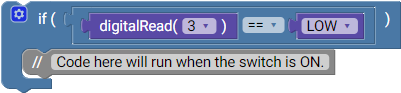``````if (digitalRead(3) == LOW)
{
// Code here will run when the switch is ON.
}
``````

If you had multiple switches, like an additional switch connected to pin 4, you could also build a more complex `if`/`else` statement like this: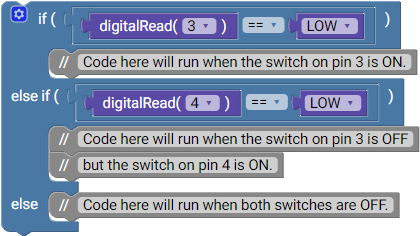``````if (digitalRead(3) == LOW)
{
// Code here will run when the switch on pin 3 is ON.
}
{
// Code here will run when the switch on pin 3 is OFF
// but the switch on pin 4 is ON.
}
else
{
// Code here will run when neither switch is ON.
}
``````

You can also "nest" `if`/`else` statements like this: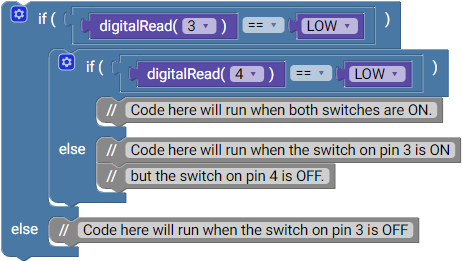``````if (digitalRead(3) == LOW)
{
{
// Code here will run when both switches are ON.
}
else
{
// Code here will run when the switch on pin 3 is ON
// but the switch on pin 4 is OFF.
}
}
else
{
// Code here will run when the switch on pin 3 is off.
}
``````

You can also combine tests together using the `&&` (AND) and `||` (OR) symbols: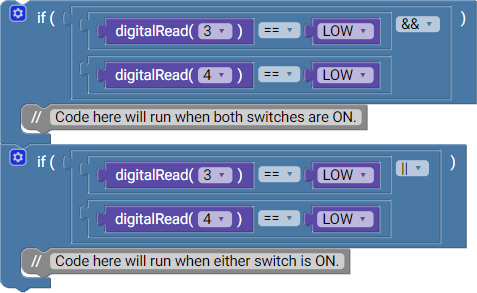``````if (digitalRead(3) == LOW && digitalRead(4) == LOW)
{
// Code here will run when both switches are ON.
}